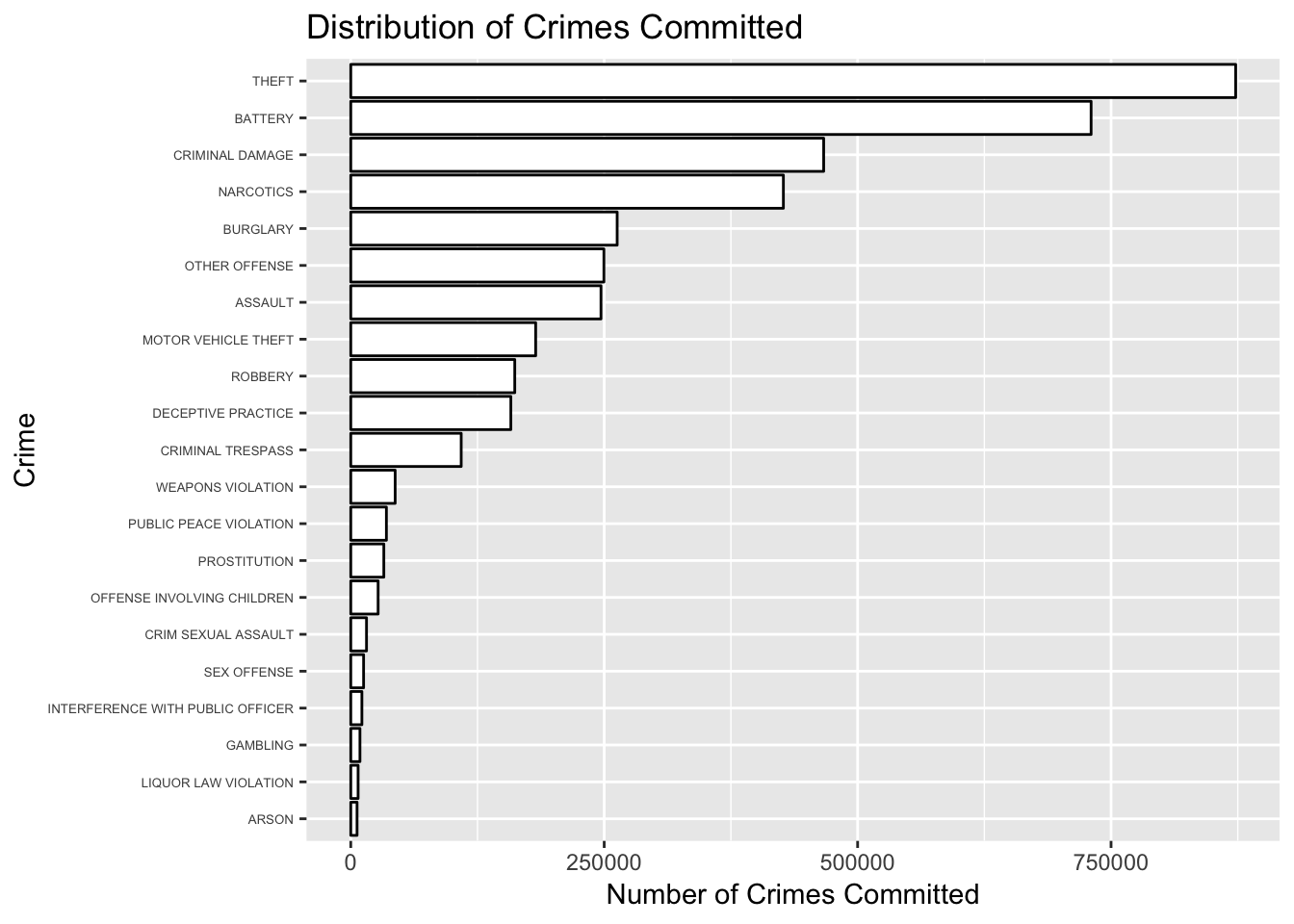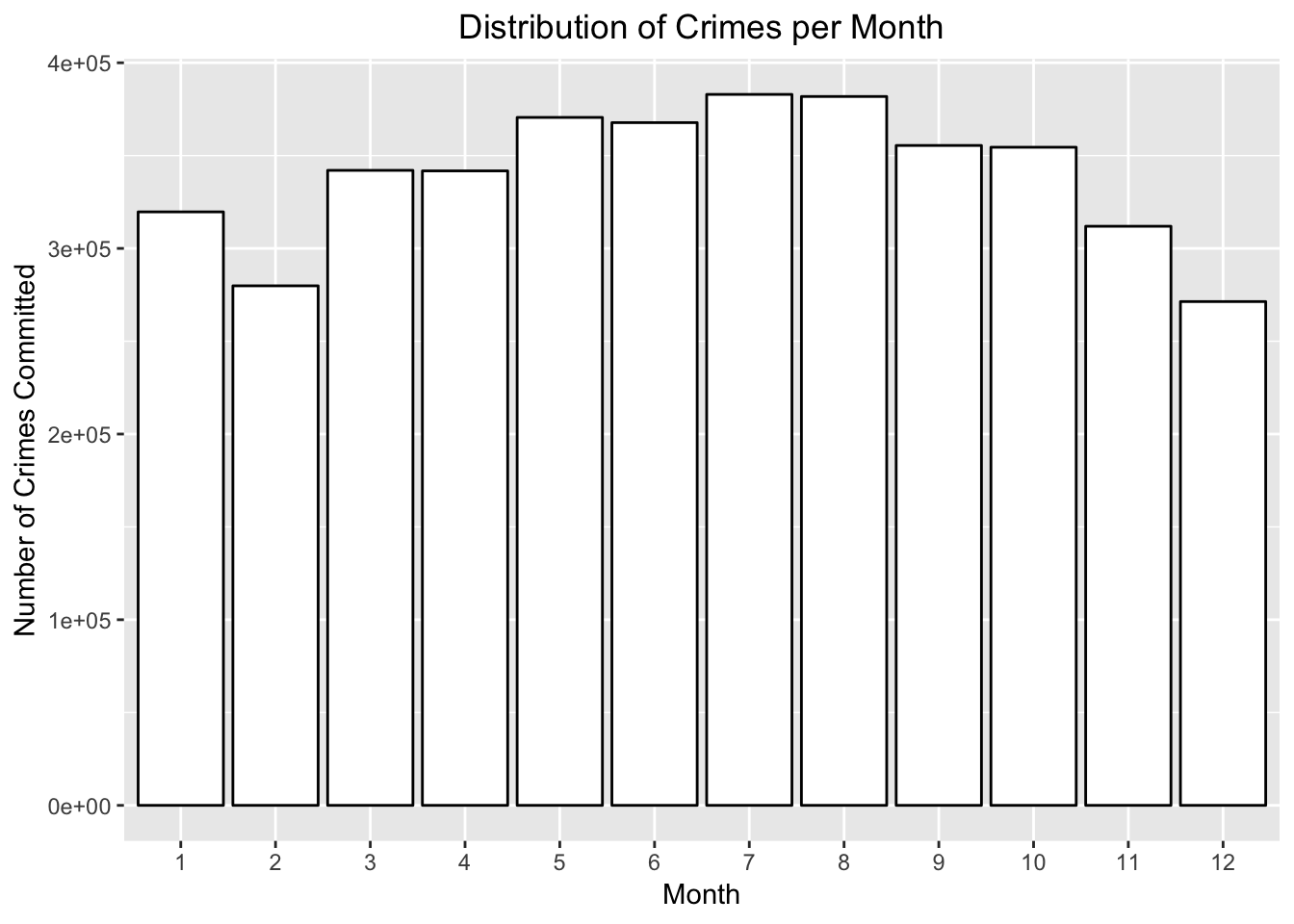# Imported Packages

``````library(tidyverse)
library(lubridate)
library(ggplot2)
library(ggmap)
library(dplyr)
library(data.table)
library(ggrepel)
library(varhandle)
library(gridExtra)``````

# Importing Data

To import all the data we read in from csv files each year range seperatly and the combine all the data with rbind to create a complete data set.

``````to2004 <- read.csv("Chicago_Crimes_2001_to_2004.csv",stringsAsFactors=FALSE)
all <- rbind(to2004,to2007,to2011,to2017)
Chicago.Aft.2008 <- rbind(to2011, to2017)``````

In addition, to plot the data we must get a map of the area of Chicago

``````register_google(key = "AIzaSyC9rrtr993vzkQlEF3HfYdzwcj0ojOnLzA")

## Cleaning an sorting data

After the data has been imported we decided to only focus on the most recent 10 years to decrease processor load on the computer. Since some of the values were imported as strings they must be transformed to numeric values before they can be graphed. Finally, for the purpose of graphing only points with a latitude and longitude can be included so we created a new dataframe with only points with a Latitude and Longitude. We also decided to create new columns using the date to help with exploratory data analysis.

``````crimes <- filter(all,Year>2007)
crimes <-filter(crimes,Year<2018)
crimes\$Longitude <- as.numeric(crimes\$Longitude)
crimes\$Latitude <- as.numeric(crimes\$Latitude)
hasLocation <- filter(crimes, !is.na(Longitude),!is.na(Latitude))
hasLocation\$Day <- factor(day(as.POSIXlt(hasLocation\$Date, format="%m/%d/%Y %I:%M:%S %p")))
hasLocation\$Month <- factor(month(as.POSIXlt(hasLocation\$Date, format="%m/%d/%Y %I:%M:%S %p")))
hasLocation\$Year <- factor(year(as.POSIXlt(hasLocation\$Date, format="%m/%d/%Y %I:%M:%S %p")))
hasLocation\$Date <- as.Date(hasLocation\$Date, "%m/%d/%Y %I:%M:%S %p")
Chicago.Aft.2008.Small <- Chicago.Aft.2008 %>% select(Date, Primary.Type, Location.Description, Arrest, Year, Latitude, Longitude)
Chicago.Aft.2008.Small <- na.omit(Chicago.Aft.2008.Small) ## removes any NA's

Chicago.Aft.2008.Small\$Latitude <- as.numeric(Chicago.Aft.2008.Small\$Latitude) ## Changes latitude to a numeric
Chicago.Aft.2008.Small\$Longitude <- as.numeric(Chicago.Aft.2008.Small\$Longitude) ## Changes latitude to a numeric
Chicago.Aft.2008.Small\$Day <- factor(day(as.POSIXlt(Chicago.Aft.2008.Small\$Date, format="%m/%d/%Y %I:%M:%S %p")))
Chicago.Aft.2008.Small\$Month <- factor(month(as.POSIXlt(Chicago.Aft.2008.Small\$Date, format="%m/%d/%Y %I:%M:%S %p")))
Chicago.Aft.2008.Small\$Year <- factor(year(as.POSIXlt(Chicago.Aft.2008.Small\$Date, format="%m/%d/%Y %I:%M:%S %p")))

Chicago.Aft.2008.Small\$Date <- as.Date(Chicago.Aft.2008.Small\$Date, "%m/%d/%Y %I:%M:%S %p")
Chicago.Aft.2008.Small <- Chicago.Aft.2008.Small[Chicago.Aft.2008.Small\$Year != "2017", ] ##exclude the year 2017
Chicago.Aft.2008.Small <- na.omit(Chicago.Aft.2008.Small) ## removes any NA's introduced by coercion``````

## Exploratory Data Analysis

Before we go about looking at the distributions of the crimes we chose, it is important to get a better understanding of the data. In order to achieve this we will be making visualizations to help us understand the data we have. First we will look at the number of crimes.

``````by_Crime <- hasLocation %>% group_by(Primary.Type)
numCrimes <- summarize(by_Crime, num = n())
View(numCrimes)
numCrimes <- numCrimes[numCrimes\$num>5000,]
## Histogram of the Crimes Committed
ggplot(numCrimes, aes(x=reorder(Primary.Type, num), num)) + geom_bar(stat = "identity",
color = "black", fill = "white") +
coord_flip() +
xlab("Crime") + ylab("Number of Crimes Committed") + ggtitle("Distribution of Crimes Committed") +
theme(
axis.text.y = element_text(vjust = .5, hjust = 1, size = 5)
)``````From this graphic we can see that theft and battery are the most common crimes. One interesting note from this graphic would be the fact that there is a category called other offense. It would be interesting to see what is in this category. However, as it contains a wide variety of crimes it would be better to not use this in our analysis. Next we will explore the number of crimes committed based upon on the month.

``````ggplot(hasLocation, aes(x=Month)) +
geom_bar(colour="black", fill="white") +
ylab('Number of Crimes Committed') + ggtitle("Distribution of Crimes per Month") +
xlab("Month") + theme(plot.title = element_text(hjust = .5))``````Interestingly, the number of crimes committed appears to peak during the summer months and sharply declines at the end of the year. This could be because during the summer months more people are out and about in Chicago and during the winter months most people elect to stay in their homes to avoid the cold. The more people being our causes more street and sidewalk crimes. One more interesting graphic to look at before we focus on only the crimes is the proportion of arresets by year and month.

``````## Colors for the gradient scale
colors <- c('navyblue', 'darkmagenta', 'darkorange1')

## Gets only the rows that resulted in arrests
arrests_only <- Chicago.Aft.2008.Small[Chicago.Aft.2008.Small\$Arrest == 'True',]
## Groups the arrests count by year and month with a total which is the count
by_arrests <- arrests_only %>% group_by(Year, Month) %>% summarise(Total = n())
## Groups by the Year and month with a total which is count
by_crime <- Chicago.Aft.2008.Small %>% group_by(Year, Month) %>% summarise(Total = n())
total <- cbind(crime_total=by_crime\$Total, by_arrests)
total\$prop <- total\$Total/total\$crime_total

prop <- ggplot(total, aes(Year, Month, fill = total\$prop)) +
geom_tile(size = 1, color = "white") + scale_fill_gradientn(colors = colors) +
geom_text(aes(label=round(total\$prop, digits = 3)), color='white', size = 3) +
labs(fill = "Proportion") +
ggtitle("Proportion of Crimes Resulting in \nArrests by Year and Month") +
theme(legend.title = element_text(hjust = .5),  ## Centered the legend title
plot.title = element_text(hjust = .5), ## Centered the plot title
panel.background = element_blank()
)
prop``````